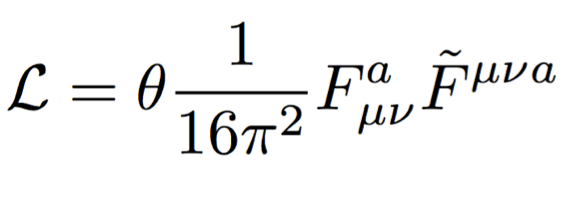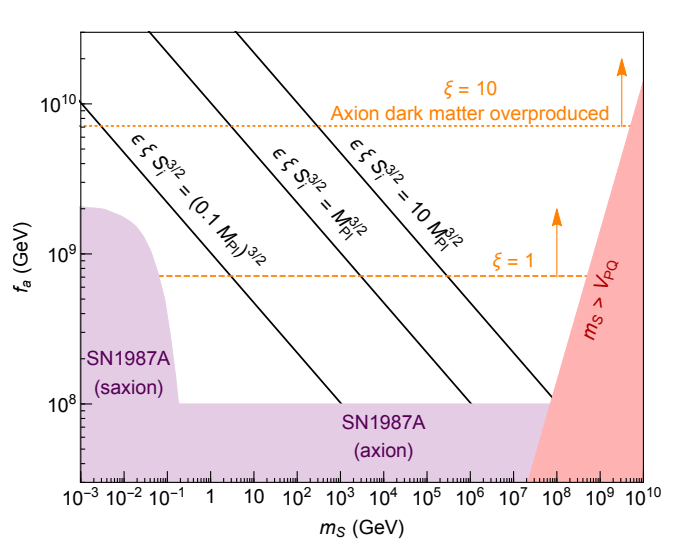# Three Birds with One Particle: The Possibilities of Axions

Title: “Axiogenesis”

Author: Raymond T. Co and Keisuke Harigaya

Reference: https://arxiv.org/pdf/1910.02080.pdf

On the laundry list of problems in particle physics, a rare three-for-one solution could come in the form of a theorized light scalar particle fittingly named after a detergent: the axion. Frank Wilczek coined this term in reference to its potential to “clean up” the Standard Model once he realized its applicability to multiple unsolved mysteries. Although Axion the dish soap has been somewhat phased out of our everyday consumer life (being now primarily sold in Latin America), axion particles remain as a key component of a physicist’s toolbox. While axions get a lot of hype as a promising dark matter candidate, and are now being considered as a solution to matter-antimatter asymmetry, they were originally proposed as a solution for a different Standard Model puzzle: the strong CP problem.

The strong CP problem refers to a peculiarity of quantum chromodynamics (QCD), our theory of quarks, gluons, and the strong force that mediates them: while the theory permits charge-parity (CP) symmetry violation, the ardent experimental search for CP-violating processes in QCD has so far come up empty-handed. What does this mean from a physical standpoint? Consider the neutron electric dipole moment (eDM), which roughly describes the distribution of the three quarks comprising a neutron. Naively, we might expect this orientation to be a triangular one. However, measurements of the neutron eDM, carried out by tracking changes in neutron spin precession, return a value orders of magnitude smaller than classically expected. In fact, the incredibly small value of this parameter corresponds to a neutron where the three quarks are found nearly in a line.The classical picture of the neutron (left) looks markedly different from the picture necessitated by CP symmetry (right). The strong CP problem is essentially a question of why our mental image should look like the right picture instead of the left. Source: https://arxiv.org/pdf/1812.02669.pdf

This would not initially appear to be a problem. In fact, in the context of CP, this makes sense: a simultaneous charge conjugation (exchanging positive charges for negative ones and vice versa) and parity inversion (flipping the sign of spatial directions) when the quark arrangement is linear results in a symmetry. Yet there are a few subtleties that point to the existence of further physics. First, this tiny value requires an adjustment of parameters within the mathematics of QCD, carefully fitting some coefficients to cancel out others in order to arrive at the desired conclusion. Second, we do observe violation of CP symmetry in particle physics processes mediated by the weak interaction, such as kaon decay, which also involves quarks.

These arguments rest upon the idea of naturalness, a principle that has been invoked successfully several times throughout the development of particle theory as a hint toward the existence of a deeper, more underlying theory. Naturalness (in one of its forms) states that such minuscule values are only allowed if they increase the overall symmetry of the theory, something that cannot be true if weak processes exhibit CP-violation where strong processes do not. This puts the strong CP problem squarely within the realm of “fine-tuning” problems in physics; although there is no known reason for CP symmetry conservation to occur, the theory must be modified to fit this observation. We then seek one of two things: either an observation of CP-violation in QCD or a solution that sets the neutron eDM, and by extension any CP-violating phase within our theory, to zero.This term in the QCD Lagrangian allows for CP symmetry violation. Current measurements place the value of at no greater than . In Peccei-Quinn symmetry, is promoted to a field.

When such an expected symmetry violation is nowhere to be found, where is a theoretician to look for such a solution? The most straightforward answer is to turn to a new symmetry. This is exactly what Roberto Peccei and Helen Quinn did in 1977, birthing the Peccei-Quinn symmetry, an extension of QCD which incorporates a CP-violating phase known as the$\theta$ term. The main idea behind this theory is to promote$\theta$ to a dynamical field, rather than keeping it a constant. Since quantum fields have associated particles, this also yields the particle we dub the axion. Looking back briefly to the neutron eDM picture of the strong CP problem, this means that the angular separation should also be dynamical, and hence be relegated to the minimum energy configuration: the quarks again all in a straight line. In the language of symmetries, the U(1) Peccei-Quinn symmetry is approximately spontaneously broken, giving us a non-zero vacuum expectation value and a nearly-massless Goldstone boson: our axion.

This is all great, but what does it have to do with dark matter? As it turns out, axions make for an especially intriguing dark matter candidate due to their low mass and potential to be produced in large quantities. For decades, this prowess was overshadowed by the leading WIMP candidate (weakly-interacting massive particles), whose parameter space has been slowly whittled down to the point where physicists are more seriously turning to alternatives. As there are several production-mechanisms in early universe cosmology for axions, and 100% of dark matter abundance could be explained through this generation, the axion is now stepping into the spotlight.

This increased focus is causing some theorists to turn to further avenues of physics as possible applications for the axion. In a recent paper, Co and Harigaya examined the connection between this versatile particle and matter-antimatter asymmetry (also called baryon asymmetry). This latter term refers to the simple observation that there appears to be more matter than antimatter in our universe, since we are predominantly composed of matter, yet matter and antimatter also seem to be produced in colliders in equal proportions. In order to explain this asymmetry, without which matter and antimatter would have annihilated and we would not exist, physicists look for any mechanism to trigger an imbalance in these two quantities in the early universe. This theorized process is known as baryogenesis.

Here’s where the axion might play a part. The$\theta$ term, which settles to zero in its possible solution to the strong CP problem, could also have taken on any value from 0 to 360 degrees very early on in the universe. Analyzing the axion field through the conjectures of quantum gravity, if there are no global symmetries then the initial axion potential cannot be symmetric . By falling from some initial value through an uneven potential, which the authors describe as a wine bottle potential with a wiggly top,$\theta$ would cycle several times through the allowed values before settling at its minimum energy value of zero. This causes the axion field to rotate, an asymmetry which could generate a disproportionality between the amounts of produced matter and antimatter. If the field were to rotate in one direction, we would see more matter than antimatter, while a rotation in the opposite direction would result instead in excess antimatter.The team’s findings can be summarized in the plot above. Regions in purple, red, and above the orange lines (dependent upon a particular constant which is proportional to weak scale quantities) signify excluded portions of the parameter space. The remaining white space shows values of the axion decay constant and mass where the currently measured amount of baryon asymmetry could be generated. Source: https://arxiv.org/pdf/1910.02080.pdf

Introducing a third fundamental mystery into the realm of axions begets the question of whether all three problems (strong CP, dark matter, and matter-antimatter asymmetry) can be solved simultaneously with axions. And, of course, there are nuances that could make alternative solutions to the strong CP problem more favorable or other dark matter candidates more likely. Like most theorized particles, there are several formulations of axion in the works. It is then necessary to turn our attention to experiment to narrow down the possibilities for how axions could interact with other particles, determine what their mass could be, and answer the all-important question: if they exist at all. Consequently, there are a plethora of axion-focused experiments up and running, with more on the horizon, that use a variety of methods spanning several subfields of physics. While these results begin to roll in, we can continue to investigate just how many problems we might be able to solve with one adaptable, soapy particle.

1. A comprehensive introduction to the strong CP problem, the axion solution, and other potential solutions: https://arxiv.org/pdf/1812.02669.pdf
2. Axions as a dark matter candidate: https://www.symmetrymagazine.org/article/the-other-dark-matter-candidate